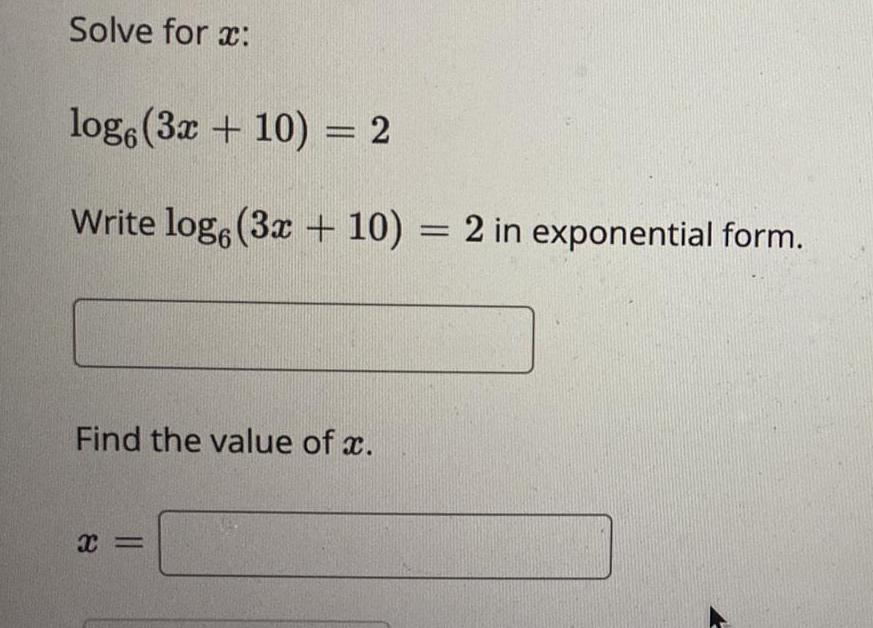Question:

# Solve for x: log6 (3x + 10) = 2 Write log6 (3x + 10) = 2 in

Last updated: 8/8/2022Solve for x: log6 (3x + 10) = 2 Write log6 (3x + 10) = 2 in exponential form. _________________ Find the value of x. x= ___________